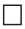Home | | Maths 5th Std | Inequality

# Inequality

The value of the expressions (6 + 3) and (6 × 3) are 9 and 18 respectively.

Inequality

The value of the expressions (6 + 3) and (6 × 3) are 9 and 18 respectively.

It means the above two expressions are not equal.

We can consider another example. Take two numbers 4 and 5. We know that 4 is not equal to 5. But, still we can relate those two numbers by a relationship.

If two expressions or numbers are not equal, one of them is greater or smaller than the other. To show ‘greater than’ and ‘lesser than’, we use the symbols ‘>’ and ‘<’ respectively.

This kind of representation is called an ‘inequality’.

Let us consider another example, the value of (9 − 5) is 4 and the value of (25 ÷ 5) is 5 and we know that 4 < 5. So, the relation between the expressions (9 − 5) and (25 ÷ 5) can be shown as (9 − 5) < (25 ÷ 5).

Note: In your higher class you will learn about two more inequalities, that is ‘≥‘ and ‘≤‘. These, two symbols can be read as, greater than or equal to (≥) and lesser than or equal to (≤).

Example 4.2

Fill in the boxes between the expressions with <, = or > as required in the following.

i) (7 +8 )(20÷2)

Solution

First, we have to add 7 and 8

7+8 = 15,

Now, we divide 20 by 2, we get

20 ÷ 2=10

Therefore, (7+8)(20 ÷ 2)

15 > 10

Hence, (7 +8) > (20 ÷ 2)

ii) (12 × 3)(9 × 4)

Solution

First, we have to multiple 12 by 3

12 × 3 = 36

Now, multiplying 9 by 4 we get,

9 × 4 = 36,

Since, (12 × 3) and (9 × 4) is equal, we have,

(12 × 3) = (9 × 4)

iii) (15−5)(8 × 3)

Solution

First, we have to subtract 5 from 15.

15−5=10

Now, multiplying 8 by 3, we get,

8 × 3 = 24

Since, (15 − 5 = 10) is less than (8 × 3 = 21), we have,

(15 − 5) < (8 × 3).

Example 4.2

Write a number in the box that will make this statements correct.

i. (6 × 4) = (−6)

Solution

The value of the expression 6 × 4 is 24. So, the number in the box has to be one that gives 24 when 6 is subtracted from it. Subtracting 6 from 30 gives 24.

Therefore, (6 × 4) = (30−6)

ii) (35÷5) < (2+)

Solution

The value of the expression 35 ÷ 5 is 7. So, the number in the box has to be such that when it is added to 2, the sum is greater than 7.

Therefore, (35 ÷ 5) < ( 2 + 6 )

Instead of 6, the solution for this condition can be 7, 8,....

Tags : Algebra | Term 3 Chapter 4 | 5th Maths , 5th Maths : Term 3 Unit 4 : Algebra
Study Material, Lecturing Notes, Assignment, Reference, Wiki description explanation, brief detail
5th Maths : Term 3 Unit 4 : Algebra : Inequality | Algebra | Term 3 Chapter 4 | 5th Maths